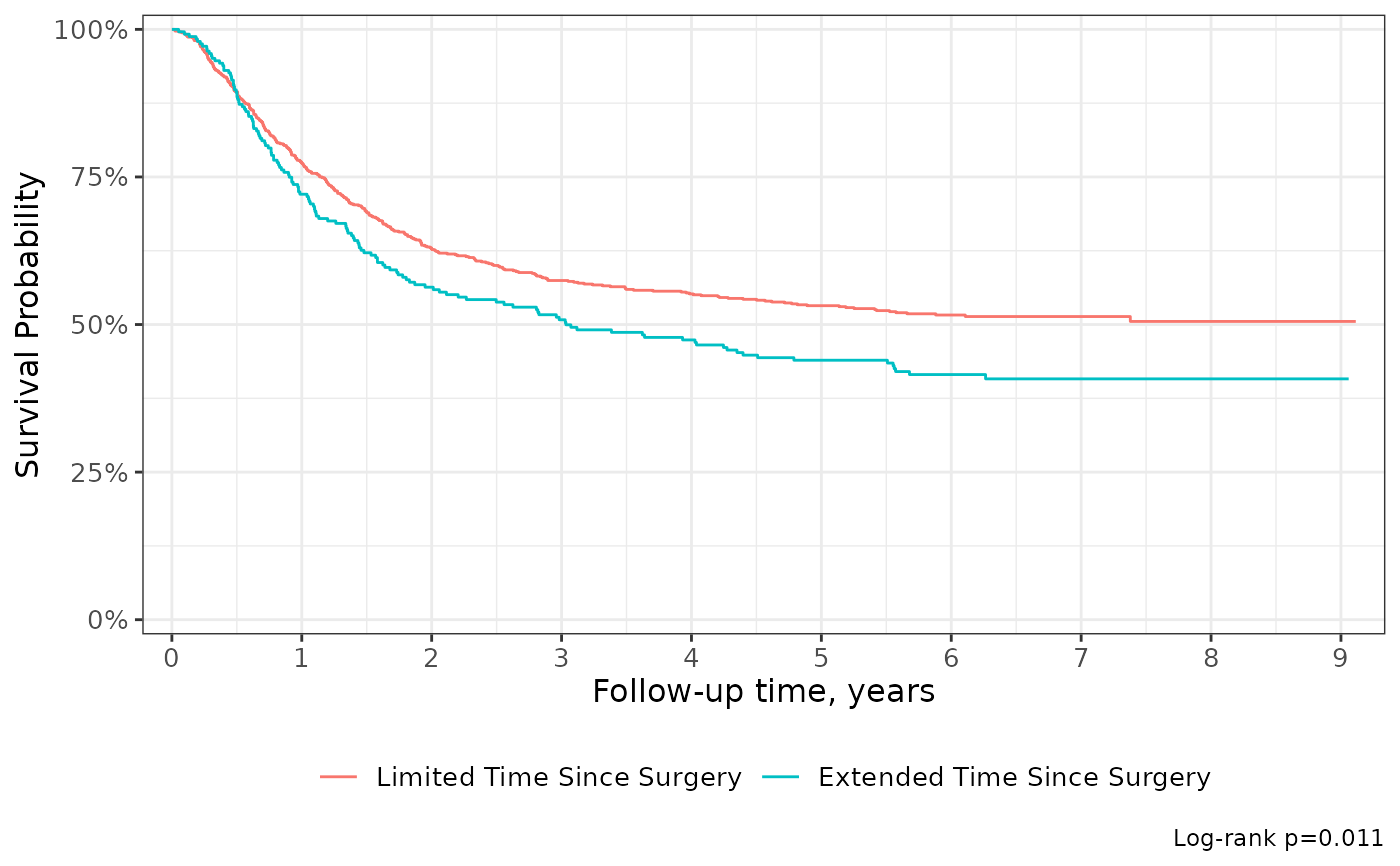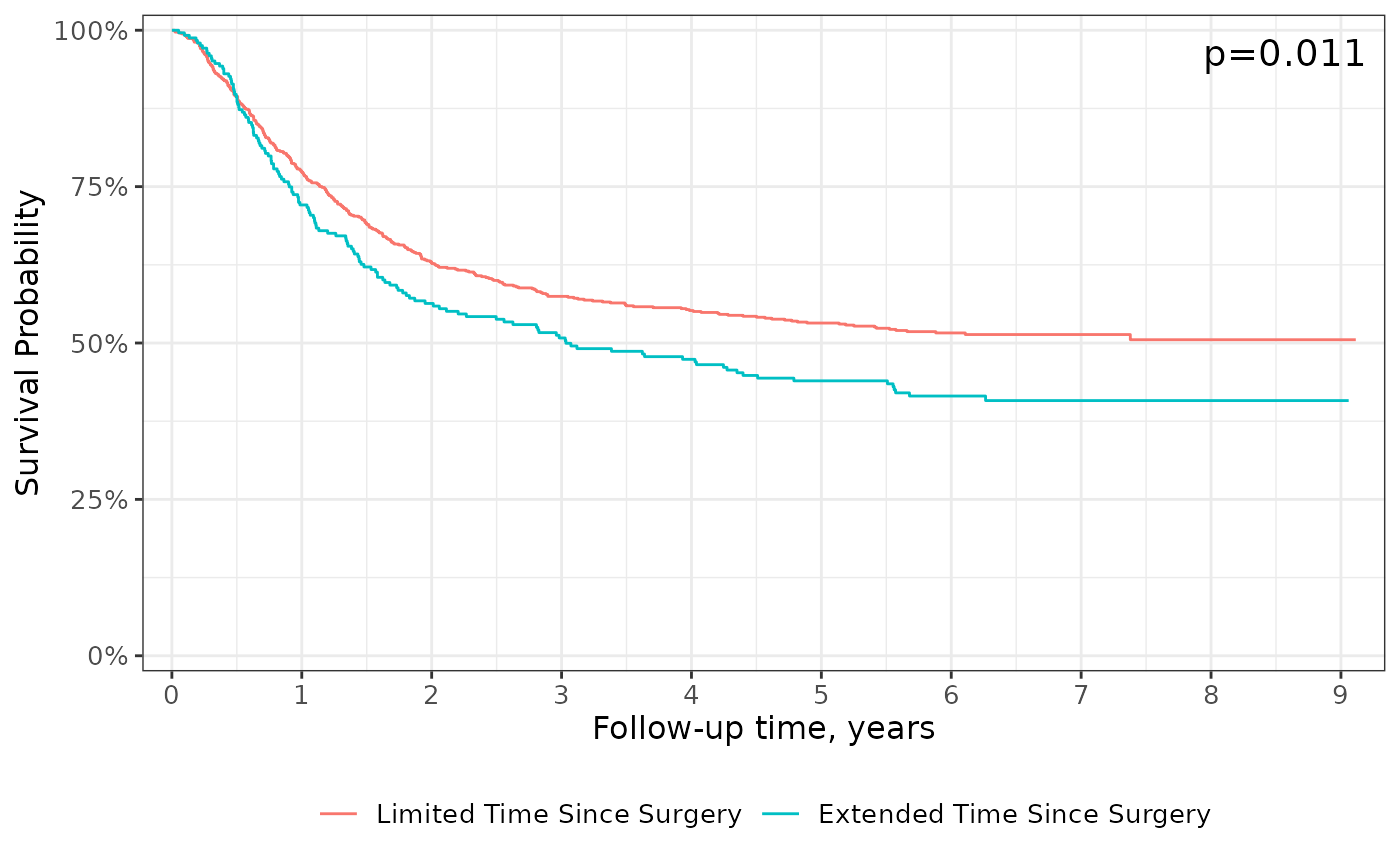• add_pvalue("caption"): Add a p-value to the figure via ggplot2::labs(caption=)

• add_pvalue("annotation"): Add a p-value text annotation via ggplot2::annotation("text")

P-values are calculated with survival::survdiff() or tidycmprsk::glance(). Examples of custom placement located in the help file for survfit_p().

When a competing risks figure includes multiple outcomes, only the p-value comparing stratum for the first outcome can be placed.

## Usage

add_pvalue(
location = c("caption", "annotation"),
caption = "{p.value}",
prepend_p = TRUE,
pvalue_fun = format_p,
rho = 0,
...
)

## Arguments

location

string indicating where to place p-value. Must be one of c("caption", "annotation")

caption

string to be placed as the caption/annotation. String will be processed with glue::glue(), and the default is "{p.value}"

prepend_p

prepend "p=" to formatted p-value

pvalue_fun

function to round and style p-value with

rho

argument passed to survival::survdiff(rho=)

...

arguments passed to ggplot2::annotate(). Commonly used arguments are x= and y= to place the p-value at the specified coordinates on the plot.

## Value

a ggplot2 figure

survfit_p()

## Examples

survfit2(Surv(time, status) ~ surg, df_colon) %>%
ggsurvfit() +